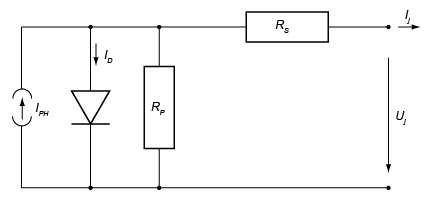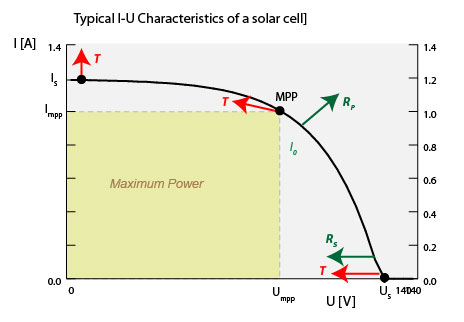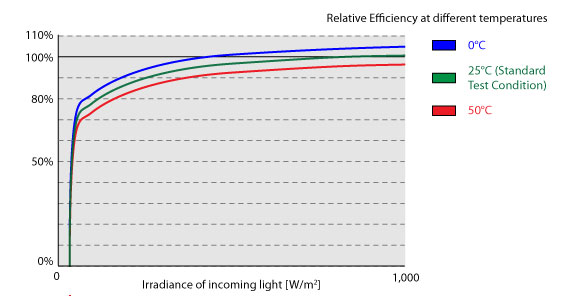Solar Power » Technologies

# Electrical Characteristics of PV Modules

## Equivalent electric circuitA solar module can be seen as a black box that with two connectors, producing a current, I, at a voltage, U.

For the purpose of the electrical characteristics of a solar cell, the inside of that black box can be described by an electric cicuit with only 4components:

1. Current Source: This is the source of the photo current, and it is:with the cell area, A, the intensity of incoming light, H, and the response factor ξ in units of A / W.
2. Diode: This non-linear element reflects the dependance on the band gap and losses to recombination. It is characterised by the reverse current, I0, which measures the leakage of electrons and re-combining and by a quality factor, q, with values between 1 - 2, an empirical factor.
3. Shunt Resistor Rp: represents losses incurred by conductors.
4. Serial Resistor Rs: also reprents losses incurred by non-ideal conductors.

The relationship between I and U of a single cell is then expressed by:with thermal voltagewith temperature, T (in Kelvin), Boltzmann constant k = 1.38e-23 and the elementary charge e = 1.602e-19. Using this formula, we can calculate maximum power points, but also behaviour under different temperatures.

In a module, a number of cells are put in series into a string, and a number of strings in parallel. Putting cells in series adds to the voltage, whereas putting cells in parallel adds to the current, so that:## Current-Voltage Curve: I-U CharacteristicsThe I-U equation can only be solved iteratively. The graph to the right shows a typical curve.

Sensitivity: The curve is highly sensitive to changes in:

 Conversion Efficiency Shift to higher currents Temperature Higher termperature results in lower open-circuit voltage, and higher short-circuit current. Overall result is a shift of the mpp to a lower power. Reverse saturation current Higher leakage results in flatter curve. Serial resistance Higher losses result in lower voltage

In practice, none of the parameters in the I-U characterisitc curve can be directrly observed. Instead, manufactureres provide the following data:

Short circuit current Current that flows when U = 0 Voltage observed when no current is flowing There is one point on the curve that provides for the maximum power output, P = U x I Provides for sensitivity to changes in temperature. This is the conversion efficiency that is observed when the module is subjected to light with intensity 1kW/m2 under standard conditions is called nominal efficiency. The peak power of the module is related to the module area A and nominal efficiency ηnomby: Ppeak= H0 η nom A   with:   H0= 1,000 W/m2 The nominal efficiency can be obtained from the manufacturer's data sheet. Should the conditions differ from the standard testing condition, the nominal module efficiency must be multiplied by a relative module efficiency, ηrel. This factor is dependant on changes in temperature, intensity of the incoming light and ratio of diffuse radiation to direct radiation. The instantaneous power supplied by the module is: Pmodule= H/H0  or:  Ppeak=H0 A ηnomηrel  with intensity of incoming light H. Values for the relative efficiency can be obtained from manufacturer's data sheet.## Relative Module Efficiency

Here is a typical curve for relative efficiency over the intensity of the incoming light for different temperatures. Naturally, at 25°C and 1,000W/m2 the relative efficiency is 1.0, as these are the standard test conditions.

Nevertheless, the conversion efficiency is nearly constant over a wide range of intensities, only dropping sharply below 10% of the standard 1,000W.

Changes in temperature cause the curve to shift upwards (if colder) or downwards (if warmer). Silicon is more sensitive to temperature changes than many of the thin-fim materials.

## Maximum Power TrackingWith light varying its intensity throughout the day, the maximum power point moves to different voltages and currents. That means, if, for instance, the voltage is forced to be constant, the solar module will most likely not operate in its power optimum.

In practice, a Maximum Power Point Tracker (MPP), is inserted between the solar module and the load (its output) in order to ensure optimum operation. Such MPP tracker is an adjustable DC- to DC transformer, which contains a high frequency switch providing a matching between the load and the solar module.

Manufacturers quote 2 efficiency factors:

1. Maximum Efficiency Factor - this is the measure of how close the device gets to the true maximum power point. Typical values are around 97%.
2. Euorpean Efficiency Factor - in reality, the tracking efficiency is not constant across the whole voltage range. However, due to variations in solar irradiance, the mpp tracker will often have to work in a non-optimal range. Hence, the European efficiency factor is an average efficiency factor that one would expect in the middle of Europe. Typical values are around 95%

Most MPP trackers are "step-down trackers", driving a high-voltage load from a low-voltage solar module. For instance:

MPP input voltage range 200 - 400V transformed into a maximum voltage of 550V.